Question

What does the triple point on a phase diagram represent? Select one: a. It represents the...

What does the triple point on a phase diagram represent?

Select one:

a. It represents the conditions under which a substance can exist in two of the three phases in stable form.

b. It represents the point at which the distinction between liquid and gas phases becomes unclear.

c. It represents the conditions of pressure and temperature under which solid, liquid, and gas all co-exist.

d. It represents the point at which the substance undergoes sublimation.

Triple point of a substance is the temperature and pressure at which the three phases of that substance coexist in thermodynamic equilibrium.

At the triple point sublimation curve , fusion curve and vaporisation curve meet.

So according to this definition a, b and d are incorrect.

Diagrammatically it can be represented as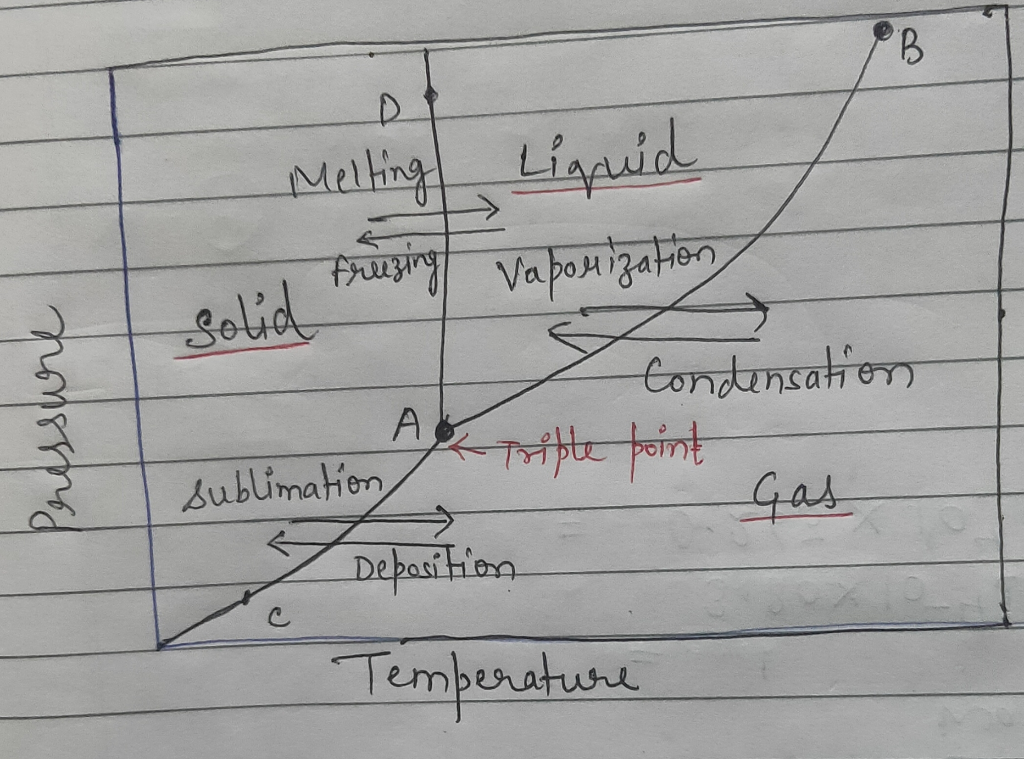Earn Coins

Coins can be redeemed for fabulous gifts.

Similar Homework Help Questions
• The triple point on a phase diagram identifies: a. The conditions in which a phase can...

The triple point on a phase diagram identifies: a. The conditions in which a phase can exist at three pressures. b. The temperature above which the supercritical fluid exists. c. The conditions in which three phases co-exist. d. The conditions in which a phase can exist at three temperatures. e. The conditions in which the melting point, freezing point, and boiling point coincide. In the phase diagram below, which is the gas to solid transition? Select one: a. A to...

• 6. Use the phase diagram below to answer the following questions. Pressure (Atmosphere) 45° 60° 100°...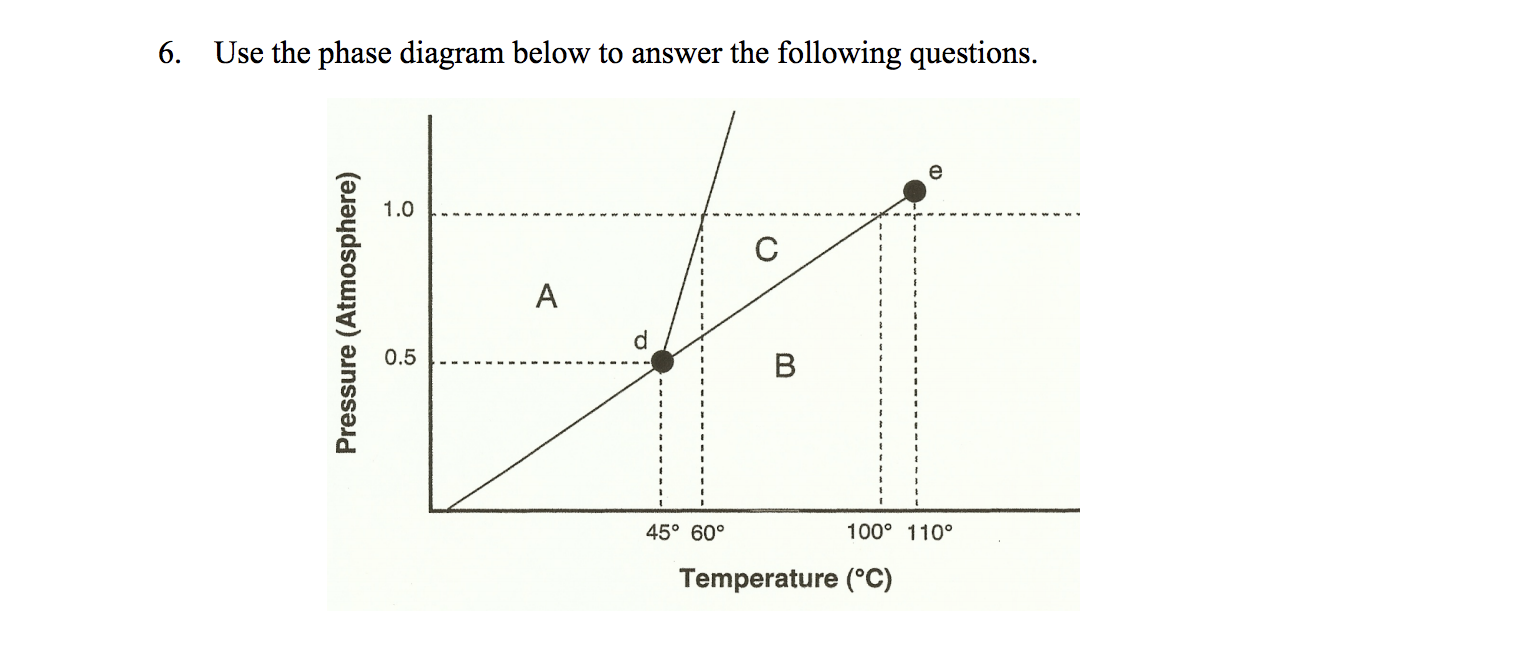6. Use the phase diagram below to answer the following questions. Pressure (Atmosphere) 45° 60° 100° 110° Temperature (°C) a. Which section represents the solid phase? b. What section represents the liquid phase? c. What section represents the gas phase? d. What letter represents the triple point? the definition of a triple point? In your own words, what is e. What is this substance's normal melting point, at 1 atmosphere of pressure? f. What is this substance's normal boiling point,...

• 9. The phase diagram for Xenon is provided opposite Liquid What phase change(s) occur as Xenon...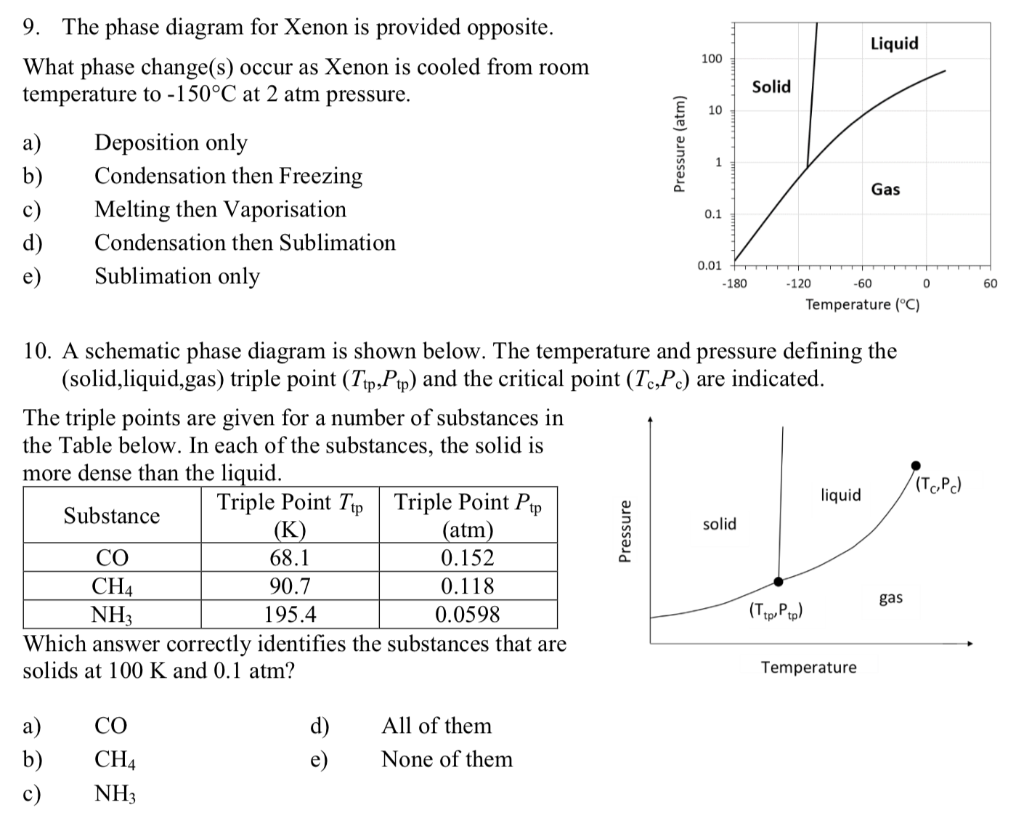9. The phase diagram for Xenon is provided opposite Liquid What phase change(s) occur as Xenon is cooled from room temperature to -150°C at 2 atm pressure 100 Solid 10 Deposition only Condensation then Freezing а) b) Gas c) Melting then Vaporisation 0.1 d) Condensation then Sublimation 0.01 Sublimation only е) -180 -120 -60 0 60 Temperature (C) 10. A schematic phase diagram is shown below. The temperature and pressure defining the (solid,liquid,gas) triple point (Tip,Pp) and the critical point...

• Consider the phase diagram of NH3. Triple point: 195.9 K and 0.069 atm Normal boiling point:...

Consider the phase diagram of NH3. Triple point: 195.9 K and 0.069 atm Normal boiling point: -33.34 oC Normal freezing point: -77.73 oC Density of liquid: 0.618g/mL Density of solid: 0.817 g/mL (a) Determine the heat of fusion, the heat of vaporization, and the heat of sublimation at the triple point. (b) Determine the entropy change of fusion, vaporization, and sublimation at the triple point. (c) Plot the phase diagram in the temperature range T: [-90 oC, 130 oC]. For...

• The diagram goes with the questions below (for Problem 7-9) On the phase diagram shown above, segment corresponds...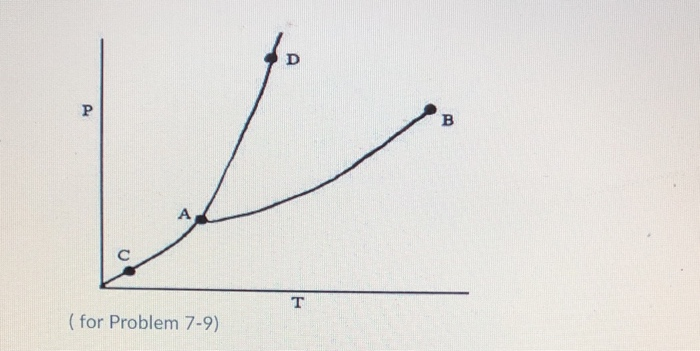The diagram goes with the questions below (for Problem 7-9) On the phase diagram shown above, segment corresponds to the conditions of temperature and pressure under which the solid and the gas of the substance are in equilibrium. 1) AB 2) AC 3) AD 4) CD 5) BC On the phase diagram shown above, the coordinates of point B corresponds to the 1) critical temperature and pressure 2) critical pressure 3) critical temperature 4) boiling point 5) triple point correspond...

• Consider the phase diagram of NH Triple point: 195.410 K and 0.06921 atm Normal boiling point:-33.342...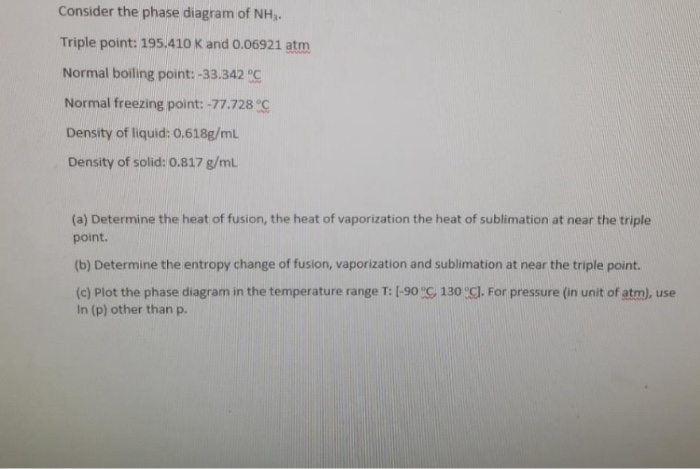Consider the phase diagram of NH Triple point: 195.410 K and 0.06921 atm Normal boiling point:-33.342 PC Normal freezing point: -77.728 °C Density of liquid: 0.618g/mL Density of solid: 0.817 g/mL (a) Determine the heat of fusion, the heat of vaporization the heat of sublimation at near the triple point. (b) Determine the entropy change of fusion, vaporization and sublimation at near the triple point. (c) Plot the phase diagram in the temperature range T: [-90 °C, 130 °C]. For...

• Select ALL statements that are True. Thank you! Select all statements that are TRUE, when considering...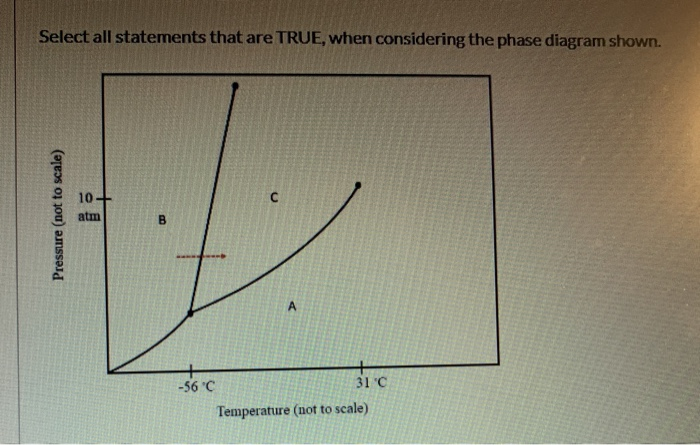Select ALL statements that are True. Thank you! Select all statements that are TRUE, when considering the phase diagram shown. 10- atm B A 31 C -56 C Temperature (not to scale) Pressure (not to scale) If the substance is heated from -40° C to 50° C, at a constant pressure of 10 atm, sublimation OCCurs Area Crepresents the liquid phase. At 10 atm of pressure, there is no temperature where the liquid phase of this substance would exist The...

• Interpretation Questions Q5: Phase diagram is a graphical representation of the equilibrium phases as a fuction...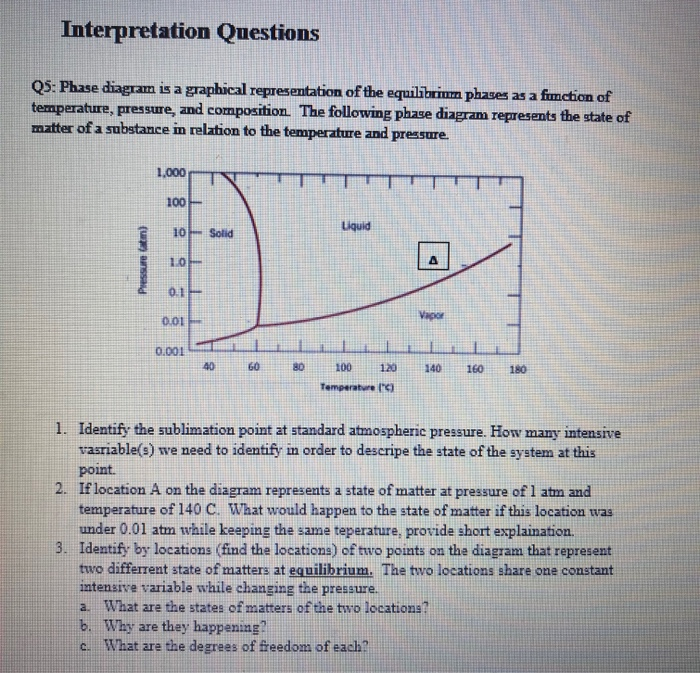Interpretation Questions Q5: Phase diagram is a graphical representation of the equilibrium phases as a fuction of temperature, pressure, and composition. The following phase diagram represents the state of matter of a substance in relation to the temperature and pressure 1.000 100 10 Solid Liquid Pressure am) 1.04 0.1 0.01 Vapor 0.001 40 60 80 100 120 140 160 180 Temperature 1. Identify the sublimation point at standard atmospheric pressure. How many intensive vasriable(s) we need to identify in order...

• GENERAL FEATURES OF A PHASE DIAGRAM; HYBRID ORBITALS AND FORMAL CHARGE MODEL 1 solid structure liquid...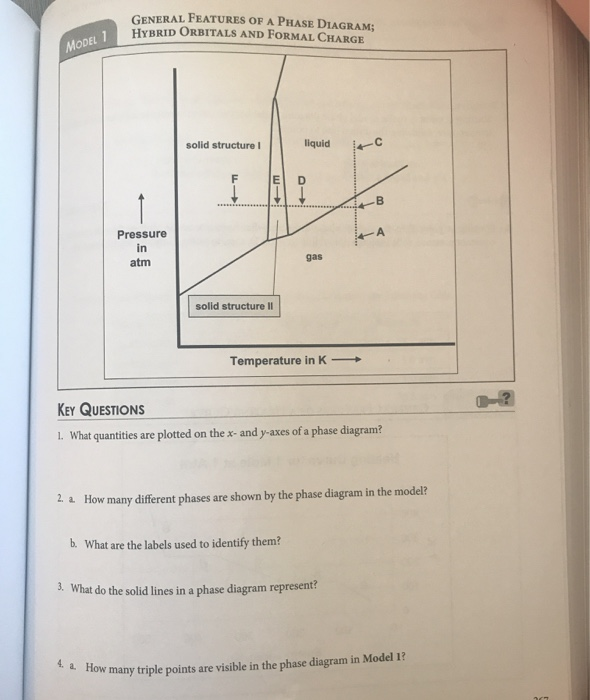GENERAL FEATURES OF A PHASE DIAGRAM; HYBRID ORBITALS AND FORMAL CHARGE MODEL 1 solid structure liquid rc Pressure in atm solid structure Il Temperature in K — KEY QUESTIONS 1. What quantities are plotted on the x-and y-axes of a phase diagram? 2. 2. How many different phases are shown by the phase diagram in the model? b. What are the labels used to identify them? 3. What do the solid lines in a phase diagram represent 4. 2. How...

• Use the data to sketch the phase diagram for xenon (Xe) and then answer the questions...

Use the data to sketch the phase diagram for xenon (Xe) and then answer the questions that follow. Normal boiling point: 165 K Normal melting point: 161 K Triple point: 152 K, .37 atm Critical temperature: 290 K Critical pressure: 57 atm A. What phase is xenon at atmospheric conditions? B. Is liquid xenon more or less dense than solid xenon? Use the diagram to justify your answer. C. What is the appropriate maximum pressure of gas that can exist...